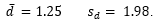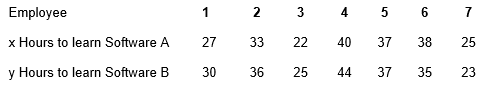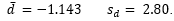# Module 8 exam 8

Problem Set 8.1

1. Independent random samples were selected from population 1 and population 2. The following information was obtained from these samples:a) Find the 95% confidence interval for estimating the difference in the population means (µ1 – µ2).

b) Can you be 95% confident that there is a difference in the means of the two populations?

2. A company would like to determine if there is a difference in the number of days that employees are absent from the East Side Plant compared to the West Side Plant. So, the company takes a sample of 54 employees from the East Side Plant and finds that these people missed an average of 5.3 days last year with a standard deviation of 1.3 days. A sample of 41 employees from the West Side plant revealed that these people were absent an average of 6.8 days last year with a standard deviation of 1.8 days.

a) Find the 96% confidence interval for estimating the difference in the population means (µ1 – µ2).

b) Can you be 96% confident that there is a difference in the means of the two populations?

c) Interpret the results.

3. The mayor of a city would like to know if there is a difference in the systolic blood pressure of those who live in her city compared to those who live in the rural area outside the city. So, 77 city dwellers are selected and it is found that their mean systolic blood pressure is 142 with a standard deviation of 10.7. Also, 65 people are selected from the surrounding rural area and it is found that their mean systolic blood pressure is 129 with a standard deviation of 8.6.

a) Find the 98% confidence interval for estimating the difference in the population means (µ1 – µ2).

b) Can you be 98% confident that there is a difference in the means of the two populations?

c) Interpret the results.

# Problem Set 8.2

Problem Set 8.2

1. Suppose we have independent random samples of size n1 = 780 and n2 = 700. The number of successes in the two samples were x1= 538 and x2 = 434. Find the 95% confidence interval for the difference in the two population proportions.

# Problem Set 8.3

Problem Set 8.3

1.The weight watchers club wants to know if there is a difference in the likelihood of a married person (whose spouse does not belong to the club) to lose weight than a single person. So, 40 married people were chosen (the only married people considered were those whose spouses did not belong to the club). Also, 40 single people were chosen. Both groups were put on the same diet. After 120 days, the average weight loss for the married group was 1 = 12.7 pounds with a standard deviation of s1=3.4 pounds. Over the same period, the average weight loss for the single group was x̄2 =11.6 pounds with a standard deviation of s2=2.5 pounds.

Using a level of significance of α=.05, is there enough evidence to indicate a difference in the mean weight lost of married people and single people?

# Problem Set 8.4

Problem Set 8.4

1. Consider the following data from a matched sample. Find the 95 % confidence interval for μd.2. Consider the following data from a matched sample. Find the 80 % confidence interval for μd.# Problem Set 8.5

Problem Set 8.5

1. Seven students take a speed reading course in order to improve their reading ability. The reading scores of the seven before and after taking the course are given below:Note that n = 7. We will define d1 = x1 – y1. After doing the appropriate calculations, we find that .Find the 80 % confidence interval for mean of the differences, μd.

2. A new drug for migraine headaches is introduced. The drug is tested on eight patients. The number of migraine headaches that each person got per year before and after taking the drug are given by:Note that n = 8. We will define , d1 = x1– y1. After doing the appropriate calculations, we find that.Find the 95 % confidence interval for mean of the differences, μd .

3. A company wants to buy new accounting software for its employees. It must decide between software A and Software B. The company will decide between the two software packages based on how quickly its employees can learn how to use the software. So, seven employees are chosen to test the software packages. The number of hours that it takes each employee to learn each software package is given by:Note that . We will define , d1 = x1 – y1. After doing the appropriate calculations, we find that.Find the 80 % confidence interval for mean of the differences, μd.

# Problem Set 8.6

Problem Set 8.6

1. Consider the following dependent random samples:a) Do hypothesis testing to see if µd < 0 at the α = .025.